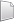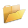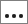# HS-4550: Multi-physics Optimization of Aircraft Radome Using HyperStudy Fit Models

In this tutorial, you will perform a multi-physics optimization using HyperStudy Fit models instead of solver models.

Before you begin, copy the model files used in this tutorial from <hst.zip>/HS-4550/ to your working directory.

The fit approximations for each physical analysis (Electromagnetic, Structural, Bird strike) have been built in prior studies and exported in portable pyfit format, which can be grouped within a single study.

## Perform Study Setup Definition

1. Start a new study in the following ways:
• From the menu bar, click File > New.
• On the ribbon, click.
2. In the Add Study dialog, enter a study name, select a location for the study, and click OK.
3. Add a HyperStudy Fit model.
1. From the Define Models step, click Add Model.
2. In the HyperStudy - Add dialog, select HyperStudy Fit model and click OK.
3. In the Label column, change the name to Structural.
4. In the Resource column, click.
5. In the HyperStudy - Load model resource dialog, select fit_Structural_analysis_OS.pyfit and click Open.
The HyperStudy Fit model is created and a Python Fit Solver is automatically populated in the Solver Execution Script column.
4. Add two more HyperStudy Fit models by repeating step 3, except change the label and resource for each model as shown in Table 1.
Table 1.
Label Resource
Electromagnetics fit_ElectroMagnetic_analysis_FEKO.pyfit
5. Click Import Variables.
In total, 14 design variables are imported: 6 variables are related to m_1, 6 are related to m_2, and 2 are related to m_3.
6. Go to the Define Input Variables step, Bounds tab and edit the first six variables as shown in Table 2.
Table 2.
Label Lower Bound Nominal Upper Bound
Thickness_Ply1 0 1.40e-04 4.2e-04
Thickness_Ply2 0 1.40e-04 4.2e-04
Thickness_Ply3 0 1.40e-04 4.2e-04
Thickness_Ply4 0 1.40e-04 4.2e-04
Thickness_Ply5 0 1.40e-04 4.2e-04
Thickness_Foam 0.001 0.004 0.012
7. For all Thickness_Ply variables, click the Modes tab and set the mode to Discrete.
8. For the Thickness_Foam variable, verify the mode is set to Continious.
9. Edit values for all Thickness_Ply variables.
1. For Thickness_Ply1, clickin the Values column.
2. For Step Size, enter 1.40e-04 and click Set.
3. Click OK.
4. Repeat step 9 for the remaining Thickness_Ply variables.
10. Link variables to ensure consistent variations in different analysis.
2. For ply1, clickin the Expression column.
3. In the Expression Builder dialog, enter m_1_m_1_varname_1*1000 in the Evaluate Expression field.
4. Repeat step 10 to define the remaing expressions as shown in Table 3.
Table 3.
Label Expression
ply2 m_1_m_1_varname_2*1000
ply3 m_1_m_1_varname_3*1000
ply4 m_1_m_1_varname_4*1000
ply5 m_1_m_1_varname_5*1000
core m_1_m_1_varname_6*1000
core_thick m_1_m_1_varname_6
plies_thick m_1_m_1_varname_1+m_1_m_1_varname_2+m_1_m_1_varname_3+m_1_m_1_varname_4+m_1_m_1_varname_5
The variables of the Bird strike and Electromagnetics models are linked to the variables of the Structural model and they will change accordingly within the evaluations.
11. Open the Constraints tab and click Add Constraint.
12. Define the constraint.
1. In the Left Expression column, click.
2. In the Expression Builder dialog, enter m_1_m_1_varname_1+m_1_m_1_varname_2+m_1_m_1_varname_3+m_1_m_1_varname_4+m_1_m_1_varname_5 in the Evaluate Expression field.
3. Click OK
4. Set Comparison to >=.
5. For Right Expression, enter 1.4e-04.
13. Go to the Test Models step.
14. Click Run Definition.
The responses are automatically extracted from the fit models.
15. Go to the Define Output Responses step, Objective/Constraints - Goals tab.
17. For Minimize, select 1 and click Add.
18. For Constraints, select 4 and click Add.
19. Define the objective and constraints as shown in Table 4.
Table 4.
Label Apply On 1 Column 2 Column
Min 1 Mass (r_2) N/A N/A
Constraint 2 TransCoeff (r_9) >= 0.97
Constraint 3 Max_Disp (r_1) <= 0.01
Constraint 4 Buckling factor (r_3) >= 2
Constraint 5 max_ply_strain_p1 (r_4) <= 0.035